Digital Lesson Solving TwoVariable Systems of Linear Equations

• Slides: 13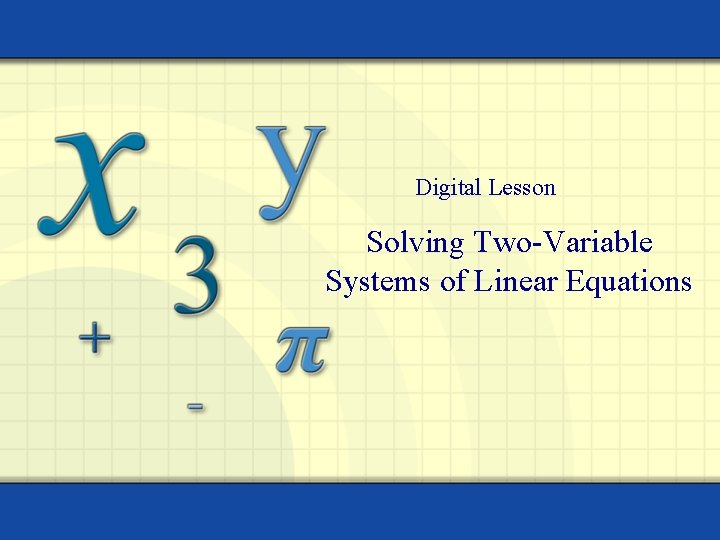Digital Lesson Solving Two-Variable Systems of Linear Equations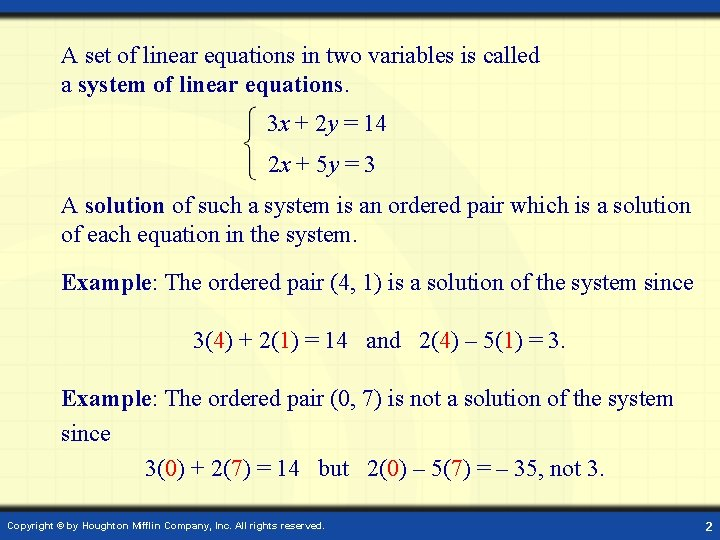A set of linear equations in two variables is called a system of linear equations. 3 x + 2 y = 14 2 x + 5 y = 3 A solution of such a system is an ordered pair which is a solution of each equation in the system. Example: The ordered pair (4, 1) is a solution of the system since 3(4) + 2(1) = 14 and 2(4) – 5(1) = 3. Example: The ordered pair (0, 7) is not a solution of the system since 3(0) + 2(7) = 14 but 2(0) – 5(7) = – 35, not 3. Copyright © by Houghton Mifflin Company, Inc. All rights reserved. 2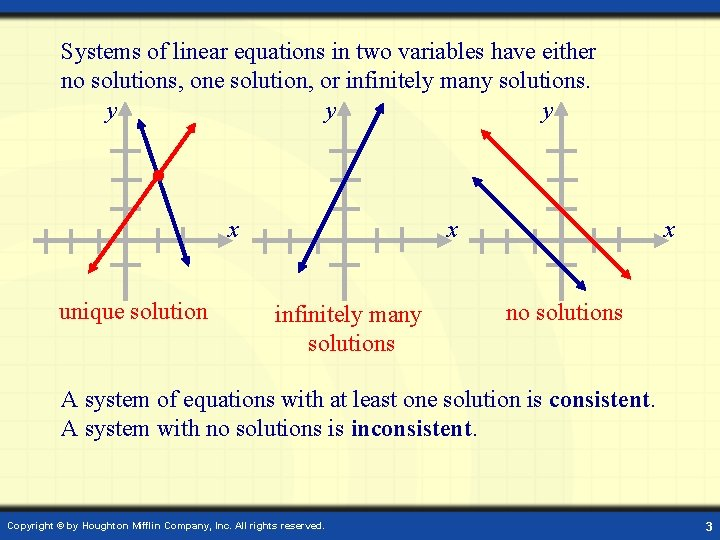Systems of linear equations in two variables have either no solutions, one solution, or infinitely many solutions. y y y x x unique solution infinitely many solutions x no solutions A system of equations with at least one solution is consistent. A system with no solutions is inconsistent. Copyright © by Houghton Mifflin Company, Inc. All rights reserved. 3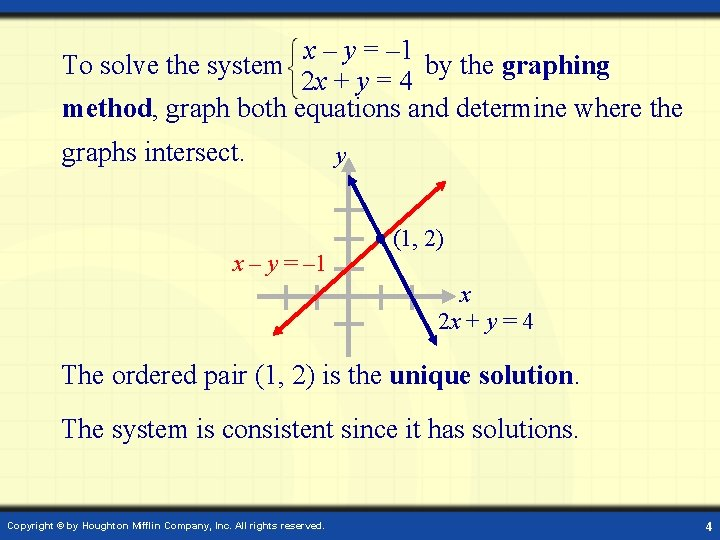x – y = – 1 To solve the system by the graphing 2 x + y = 4 method, graph both equations and determine where the graphs intersect. x – y = – 1 y (1, 2) x 2 x + y = 4 The ordered pair (1, 2) is the unique solution. The system is consistent since it has solutions. Copyright © by Houghton Mifflin Company, Inc. All rights reserved. 4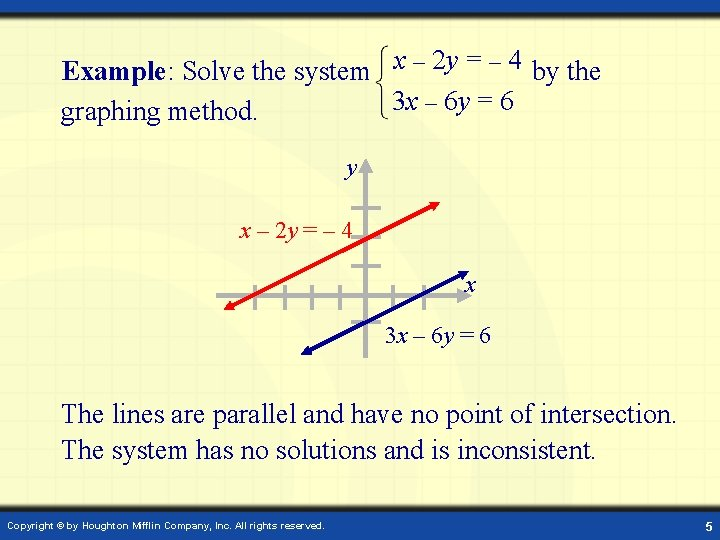Example: Solve the system x – 2 y = – 4 by the 3 x – 6 y = 6 graphing method. y x – 2 y = – 4 x 3 x – 6 y = 6 The lines are parallel and have no point of intersection. The system has no solutions and is inconsistent. Copyright © by Houghton Mifflin Company, Inc. All rights reserved. 5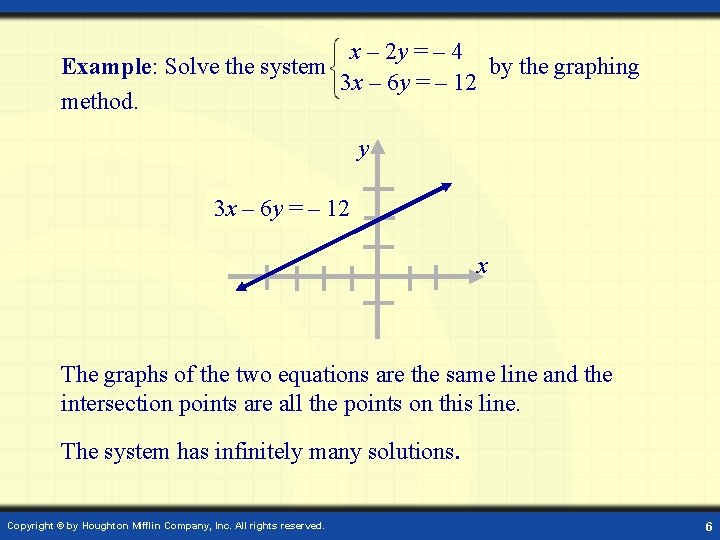x – 2 y = – 4 Example: Solve the system by the graphing 3 x – 6 y = – 12 method. y 2 y == –– 12 4 3 xx –– 6 y x The graphs of the two equations are the same line and the intersection points are all the points on this line. The system has infinitely many solutions. Copyright © by Houghton Mifflin Company, Inc. All rights reserved. 6To solve a system by the substitution method: 1. Select an equation and solve for one variable in terms of the other. 2. Substitute the expression resulting from Step 1 into the other equation to produce an equation in one variable. 3. Solve the equation produced in Step 2. 4. Substitute the value for the variable obtained in Step 3 into the expression obtained in Step 2. 5. Check the solution. Copyright © by Houghton Mifflin Company, Inc. All rights reserved. 7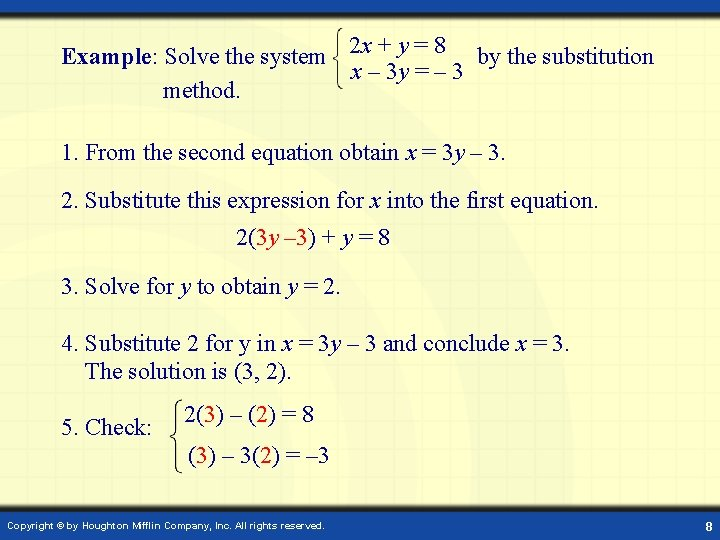Example: Solve the system 2 x + y = 8 by the substitution x – 3 y = – 3 method. 1. From the second equation obtain x = 3 y – 3. 2. Substitute this expression for x into the first equation. 2(3 y – 3) + y = 8 3. Solve for y to obtain y = 2. 4. Substitute 2 for y in x = 3 y – 3 and conclude x = 3. The solution is (3, 2). 5. Check: 2(3) – (2) = 8 (3) – 3(2) = – 3 Copyright © by Houghton Mifflin Company, Inc. All rights reserved. 8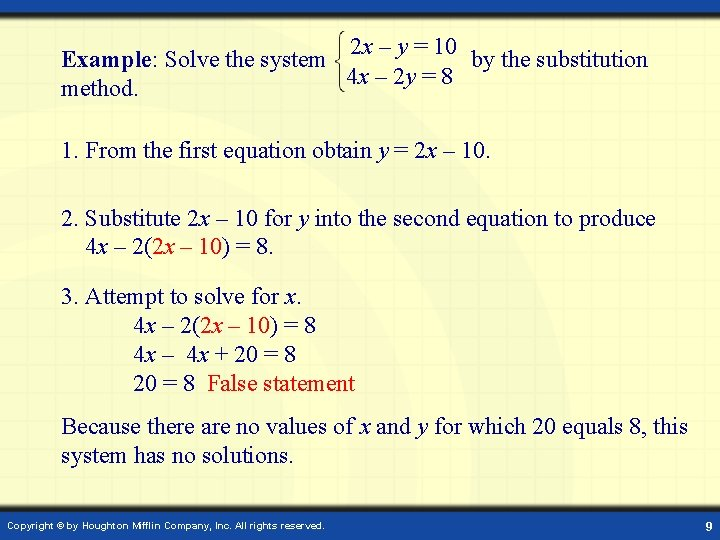2 x – y = 10 Example: Solve the system by the substitution 4 x – 2 y = 8 method. 1. From the first equation obtain y = 2 x – 10. 2. Substitute 2 x – 10 for y into the second equation to produce 4 x – 2(2 x – 10) = 8. 3. Attempt to solve for x. 4 x – 2(2 x – 10) = 8 4 x – 4 x + 20 = 8 False statement Because there are no values of x and y for which 20 equals 8, this system has no solutions. Copyright © by Houghton Mifflin Company, Inc. All rights reserved. 9To solve a system by the addition (or elimination) method: 1. Multiply either or both equations by nonzero constants to obtain opposite coefficients for one of the variables in the system. 2. Add the equations to produce an equation in one variable. Solve this equation. 3. Substitute the value of the variable found in Step 2 into either of the original equations to obtain another equation in one variable. Solve this equation. 4. Check the solution. Copyright © by Houghton Mifflin Company, Inc. All rights reserved. 10Example: Solve the system 5 x + 2 y = 11 by the addition method. 3 x + 4 y = 15 1. Multiply the first equation by – 2 to make the coefficients of y opposites. – 10 x – 4 y = – 22 3 x + 4 y = 15 2. Add the equations to obtain – 7 x = – 7. Therefore x = 1. 3. Substitute 1 for x in the first equation to produce 5(1) + 2 y = 11 2 y = 6 Therefore y = 3. The solution is (1, 3). 4. Check: 5(1) + 2(3) = 11 3(1) + 4(3) = 15 Copyright © by Houghton Mifflin Company, Inc. All rights reserved. 11Example: A plane with a tailwind flew 1920 mi in 8 hours. On the return trip, against the wind, the plane flew the same distance in 12 hours. What is the speed of the plane in calm air and the speed of the tailwind? Let x = speed of the plane in calm air y = speed of the tailwind Use the formula Rate × Time = Distance. Rate With Wind x+y Against Wind x-y Time 8 12 Distance 8(x + y) 12(x - y) This yields a system of equations in x and y. Use the addition method to solve the system. Copyright © by Houghton Mifflin Company, Inc. All rights reserved. 8 x + 8 y = 1920 12 x – 12 y = 1920 Example continues 128 x + 8 y = 1920 Example continued: Solve the system 12 x – 12 y = 1920 using the addition method. 1. Multiply the first equation by 3 and the second equation by 2. 3(8 x + 8 y) = 3(1920) 2(12 x – 12 y) = 2(1920) 24 x + 24 y = 5760 24 x – 24 y = 3840 2. Add the equations to obtain 48 x = 9600. Therefore x = 200. 3. Substitute 200 for x in the first equation. y = 40 8(200) + 8 y = 1920 4. In 8 hours with an airspeed of 240 mph the plane will travel 8 × 240 = 1920 mi on the first leg of its flight. In 12 hours with an airspeed of 160 mph the plane will travel 12 × 160 = 1920 on the return leg of its flight. Copyright © by Houghton Mifflin Company, Inc. All rights reserved. 13